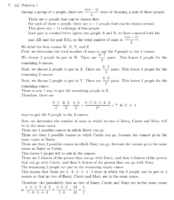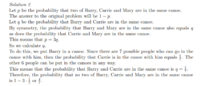# Help with probability question

#### Ed9630

##### New member
Hello,

This is my very first post on this site, so forgive me if I have committed any taboos.
This is a question from the 2018 University of Waterloo Euclid contest.

The Question
Eight people, including triplets Barry, Carrie, and Mary, are going for a trip in four canoes. Each canoe seats two people. The eight people are to be randomly assigned to the four canoes in pairs. What is the probability that no two of Barry, Carrie and Mary will be in the same canoe?

My Solution
Let S be the total number of ways to assign the eight people to the four canoes.
Let A be the event that no two of Barry, Carrie and Mary are in the same canoe.

n(S)=$$\displaystyle {8 \choose 2}{6 \choose 2}{4 \choose 2}{2 \choose 2}$$
=(28)(15)(6)(1)
=2520

n(A')=number of ways two of Barry, Carrie and Mary are in the same canoe
=$$\displaystyle {3 \choose 2}{2 \choose 2}{6 \choose 2}{4 \choose 2}{2 \choose 2}$$
=(3)(1)(15)(6)(1)
=270

My reasoning for this step was that out of the three triplets, I am choosing two of them ($$\displaystyle {3 \choose 2}$$). Then, the two that were chosen are going to be in the same canoe ($$\displaystyle {2 \choose 2}$$). Then, the remaining 6 people are put into the remaining three canoes ($$\displaystyle {6 \choose 2}{4 \choose 2}{2 \choose 2}$$).

Therefore,
P(A)=[n(S)-n(A')] / n(S)
=(2520-270) / 2520
=2250/2520
=25/28

Solution in the Solutions Manual
Two different solutions are provided in the solutions manual, both of which yield a solution of 4/7. I have attached them both below.Both of these solutions are different from what I have done, but they also seem logical. I am assuming that there is a flaw in my reasoning somewhere in my solution, but I can't seem to figure out where I have gone wrong.

#### Attachments

• 170.5 KB Views: 0

#### Romsek

##### Full Member
We don't care about the order of the canoes. So the total number of arrangements is just

$$\displaystyle \dfrac{\dbinom{8}{2}\dbinom{6}{2}\dbinom{4}{2}}{4!} = 105$$

I don't understand what you've done for the probability that they are in separate canoes.

Then the number of ways the 3 of them can be in 3 separate canoes is

$$\displaystyle \dbinom{5}{1}\dbinom{4}{1}\dbinom{3}{1}=60$$

Having chosen those 3 people the two in the last canoe are fixed.

$$\displaystyle p = \dfrac{60}{105}=\dfrac{4}{7}$$

•Ed9630

#### Ed9630

##### New member
We don't care about the order of the canoes. So the total number of arrangements is just

$$\displaystyle \dfrac{\dbinom{8}{2}\dbinom{6}{2}\dbinom{4}{2}}{4!} = 105$$

I don't understand what you've done for the probability that they are in separate canoes.

Then the number of ways the 3 of them can be in 3 separate canoes is

$$\displaystyle \dbinom{5}{1}\dbinom{4}{1}\dbinom{3}{1}=60$$

Having chosen those 3 people the two in the last canoe are fixed.

$$\displaystyle p = \dfrac{60}{105}=\dfrac{4}{7}$$
That makes sense. I see where I went wrong now.

Thanks a lot!